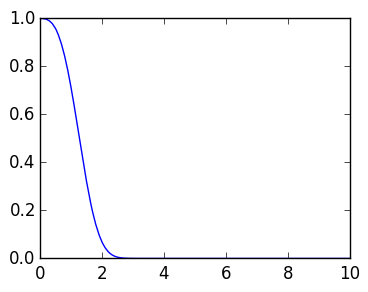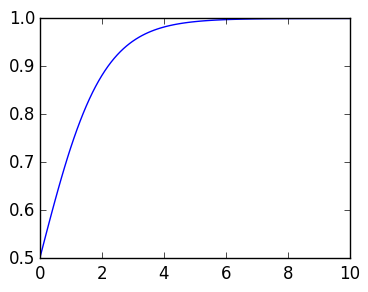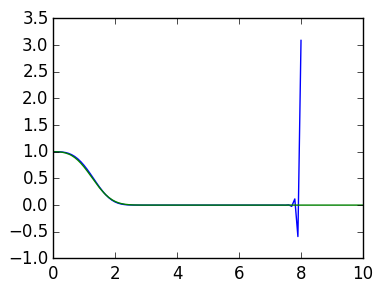# Lecture 33: Linear ODEs¶

We now turn our attention to the numerical solution of ordinary differential equations. We will focus on linear ODEs to begin with. The reason we do this is it allows us to view the numerical solution as reducing infinite-dimensional linear equations (the ODE) to finite-dimensional linear systems (matrices).

To emphasize the difference between linear and nonlinear, consider the following two examples:

1. Example of a linear ODE: $$y'(t) - a(t) y(t) =0, y(0)=1$$ Write this as $Ly = 0, y(0)=1$ where $L$ is a linear operator: $$L = D - a(t)$$ where $D$ is the derivative operator. This is called a linear operator because, for constant $c_1$ and $c_2$, we have $$L(c_1 y_1 + c_2 y_2) = c_1 L y_1 + c_2 L y_2 = c_1 (y_1' -a(t) y_1) + c_2(y_2' -a (t) y_2)$$
1. Example of a nonlinear ODE: the following logistic equation is a nonlinear ODE, since it depends quadratically on $y$:
$$y'- y(1-y) = 0, y(0) =0.5$$

## Solving ode45¶

When the equation is an initial value problem, we can solve both linear and nonlinear ODEs using ode45. Here is an example of a linear ODE:

In :
using ODE, PyPlot

a=t->-t^2
t,y=ode45((t,y)->a(t)*y,1.,0.:0.1:10.)
plot(t,y);Similarly, we can solve a nonlinear ODE:

In :
t,y=ode45((t,y)->y*(1-y),0.5,0.:0.1:10.)
plot(t,y);## Boundary value problems¶

The distinction between linear and nonlinear becomes greater when we consider other types of problems. The following is a linear intial value problem, since the constraints are specified at a single point, $t=0$:

$$u'' -a(t)u = 0, u(0) = 1, u'(0)=0$$

While we can solve this with ode45 by rewriting as a system, we can also solve it in ApproxFun:

In :
using ApproxFun

D=Derivative()   # the derivative operator
B=ivp()   # conditions corresponding to u(0),u(1)
a=Fun(t->-t^2,[0.,10.])
u=[B;D^2-a]\[1.,0.]
ApproxFun.plot(u)

Out:

However, many problems are not initial value problems, but rather boundary value problem. One can imagine this coming up if, say, you observe a bullet at two different points, and know it solves an ODE, and want to recover the solution.

As a simple example, we consider

$$u'' -a(t)u = 0, u(0) = 1, u(10)=0.5$$

Because it's linear, we can solve BVPs just as easily as initial value problems:

In :
D=Derivative()
B=dirichlet()
a=Fun(t->t^2,[0.,10.])
u=[B;D^2-a]\[1.,0.5]
ApproxFun.plot(u)

Out:

# Euler's method¶

Our starting point is to construct Euler's method for the ODE

$$y' = f(t,y), y(0) =1$$

The motivation is the observation that

$$y(h) = y(0) + hy'(0) + {h^2 \over 2} y''(\chi) = y(0) + h y'(0) + O(h^2) = y(0) + h f(0,y(0)) + O(h^2)$$

where $h$ is a small step. Thus we have $y(h) \approx y(0) + h f(0,y(0))$, and more generally, $y(t_{k+1}) \approx y(t_k) + h f(t_k,y(t_k))$ for $t_k \triangleq k*h$. We use this to construct an approximation $w_k \approx y(t_k)$ via:

$$w_{k+1} = w_k + h f(t_k,w_k)$$

For the linear ODE $$y'(t) = a(t) y(t)$$, this becomes

$$w_0 = y(0), w_{k+1} = (1 + h a(t_k)) w_k$$

We observe that this does indeed approximate the solution, by comparing to ode45:

In :
a=t->-t^2

# step 1: choose a time step
h=0.1
n=round(Int,8/h)
w=zeros(n+1)  # a vector that approximates y at each time step

t=0.:h:10.

w=1.
M=length(w)-1
for k=1:M
w[k+1]=(1 + h*a(t[k]))*w[k]
end

plot(t[1:M+1],w[1:M+1])

t,y=ode45((t,y)->a(t)*y,1.,t)
plot(t,y);Taking a small step size and we get an accurate approximation, though it eventually blows up.

The key observation we make now is that we are in fact solving a lower triangular linear system with forward elimination:

$$\begin{pmatrix} 1 \cr 1+ha(t_0) & -1 \cr & 1+ha(t_1) & -1 \cr && 1+ha(t_2) & -1 \cr &&&\ddots & \ddots \end{pmatrix} \begin{pmatrix} w_0 \cr w_1 \cr w_2 \cr w_3 \cr \vdots \end{pmatrix} = \begin{pmatrix} 1 \cr 0 \cr 0 \cr 0 \cr \vdots \end{pmatrix}$$